R script: survival.R

Mlxtran code: model/survival1.txt ; model/survival2.txt

$$\newcommand{\esp}{\mathbb{E}\left(#1\right)} \newcommand{\var}{\mbox{Var}\left(#1\right)} \newcommand{\deriv}{\dot{#1}(t)} \newcommand{\prob}{ \mathbb{P}\!(#1)} \newcommand{\eqdef}{\mathop{=}\limits^{\mathrm{def}}} \newcommand{\by}{\boldsymbol{y}} \newcommand{\bc}{\boldsymbol{c}} \newcommand{\bpsi}{\boldsymbol{\psi}} \def\pmacro{\texttt{p}} \def\like{{\cal L}} \def\llike{{\cal LL}} \def\logit{{\rm logit}} \def\probit{{\rm probit}} \def\one{{\rm 1\!I}} \def\iid{\mathop{\sim}_{\rm i.i.d.}} \def\simh0{\mathop{\sim}_{H_0}} \def\df{\texttt{df}} \def\res{e} \def\xomega{x} \newcommand{\argmin}{{\rm arg}\min_{#1}} \newcommand{\argmax}{{\rm arg}\max_{#1}} \newcommand{\Rset}{\mbox{\mathbb{R}}} \def\param{\theta} \def\setparam{\Theta} \def\xnew{x_{\rm new}} \def\fnew{f_{\rm new}} \def\ynew{y_{\rm new}} \def\nnew{n_{\rm new}} \def\enew{e_{\rm new}} \def\Xnew{X_{\rm new}} \def\hfnew{\widehat{\fnew}} \def\degree{m} \def\nbeta{d} \newcommand{\limite}{\mathop{\longrightarrow}\limits_{#1}} \def\ka{k{a}} \def\ska{k{\scriptscriptstyle a}} \def\kel{k{e}} \def\skel{k{\scriptscriptstyle e}} \def\cl{C{\small l}} \def\Tlag{T\hspace{-0.1em}{lag}} \def\sTlag{T\hspace{-0.07em}{\scriptscriptstyle lag}} \def\Tk{T\hspace{-0.1em}{k0}} \def\sTk{T\hspace{-0.07em}{\scriptscriptstyle k0}} \def\thalf{t{1/2}} \newcommand{\Dphi}{\partial_\pphi #1} \def\asigma{a} \def\pphi{\psi} \newcommand{\stheta}{{\theta^\star}} \newcommand{\htheta}{{\widehat{\theta}}}$$

# 1 Introduction

Here, observations are the times at which events occur.’’ An event may be one-off (e.g., death, hardware failure) or repeated (e.g., epileptic seizures, mechanical incidents, strikes).

To begin with, we will consider a one-off event. The survival function $$S(t)$$ gives the probability that the event happens after time $$t>t_{\rm start}$$: $S(t) \ \ \eqdef \ \ \prob{T>t} .$

The hazard function $$h(t)$$ is defined for individual $$i$$ as the instantaneous rate of the event at time $$t$$, given that the event has not already occurred: $h(t) \ \ \eqdef \ \ \lim_{dt\to 0} \frac{S(t) - S(t + dt)}{ S(t) \, dt} .$ This is equivalent to $h(t) \ \ = \ \ -\frac{d}{dt} \log{S(t)} .$

Depending on the application, the length of time to this event may be called the survival time or the failure time. In general, we simply say time-to-event.

The random variable representing the time-to-event is typically written $$T$$. There are then several ways to define observations, depending on the situation:

• The event time is exactly observed at time $$t$$. Here, observation is $$T = t$$’’.

The distribution of time to event data is defined in the block DEFINITIONof the Section [LONGITUDINAL] by the hazard function $$h$$ previously defined in the block EQUATION. This is the Mlxtran code for a single exactly observed event:

DEFINITION:
e = {type = event,
maxEventNumber = 1,
hazard = h}

• We may know the event has happened in an interval $$I$$ of length $$L$$ but not know the exact time. This is interval censoring and observation is the event: $$T \in I$$’’.
DEFINITION:
e = {type = event,
maxEventNumber = 1,
eventType = intervalCensored,
intervalLength = L,
hazard = h}

• If we assume that the trial ends at time $$t_{\rm stop}$$, the event may happen after the end. This is right censoring. Here, observation is the event: $$T > t_{\rm stop}$$’’.
DEFINITION:
e = {type = event,
maxEventNumber = 1,
rightCensoringTime = tstop,
hazard = h}


Remark: it is possible to combine interval censoring and right censoring.

Let us now consider repeated events. For any given hazard function $$h$$, the survival function $$S$$ now represents the survival since the previous event at $$t_{j-1}$$, given here in terms of the cumulative hazard from $$t_{j-1}$$ to $$t_{j}$$: \begin{align} S(t_{j} | t_{j-1}) &= \prob{T_{j} > t_{j}\,|\,T_{j-1} = t_{j-1}} \\ &= \exp\left({-\int_{t_{j-1}}^{t_{j}} h(u) \, du}\right) \end{align}

Taking into account censoring for repeated events is slightly different from one-off events: let $$(b_{0}, b_1], (b_{1}, b_2], \ldots , (b_{K-1}, b_K]$$ be a sequence of successive intervals with $t_{\rm start}=b_0<b_1<b_2<\ldots <b_K=t_{\rm stop}.$ We do not know the exact event times, but a sequence $$(m_{k};\,1 \leq k \leq K)$$} is observed where $$m_{k}$$ is the number of events that occurred in interval $$(b_{k-1}, b_k]$$.

The Mlxtran code for repeated events is the same as for the single case, without the statement maxEventNumber = 1 if the number of events is not bounded, or with maxEventNumber = M if the maximum number of possible events is $$M$$.

Remark: if the number of events is not bounded, it is mandatory to define a right censoring time for simulation.

# 2 Simulation of a single event

We consider a Weibull model for a single event in this first example: \begin{align} h(t) &= \frac{k}{\lambda} \left(\frac{t}{\lambda}\right)^{k-1}, \\ S(t) &= e^{-(t/\lambda)^{k}} \end{align} The study stops at time 60: there is therefore a right censoring time equal to 60.

This model is implemented in the file survival1.txt:

[LONGITUDINAL]
input = {beta,lambda}

EQUATION:
h=(beta/lambda)*(t/lambda)^(beta-1)

DEFINITION:
e = {type               = event,
maxEventNumber     = 1,
rightCensoringTime = 60,
hazard             = h}


We will use simulx for computing $$h$$ every hour between 0h and 60h and for generating a single event starting at time 0, with $$\beta=1.5$$ and $$\lambda=20$$. It is mandatory to state explicitly when the experiment starts (at time $$t=0$$ in this example), defining time=0 for the output e.

p <- c(beta = 1.5, lambda=20)
h <- list(name='h', time=seq(0, 60, by=0.2))
e <- list(name='e', time=0)

res <- simulx(model     = 'model/survival1.txt',
settings  = list(seed=123),
parameter = p,
output    = list(h, e))

Information about the simulated event is stored in the data frame res$e print(res$e)
##       time e
## 1  0.00000 0
## 2 28.81942 1

The first line means that the experiment started at time 0 (i.e., the event happened after $$t=0$$), the second line means that the event was observed at time $$t=28.82$$.

The hazard function is stored in the data frame res$h hazard <- res$h
plot(x=hazard$time, y=hazard$h, type='l', xlab="time", ylab="hazard")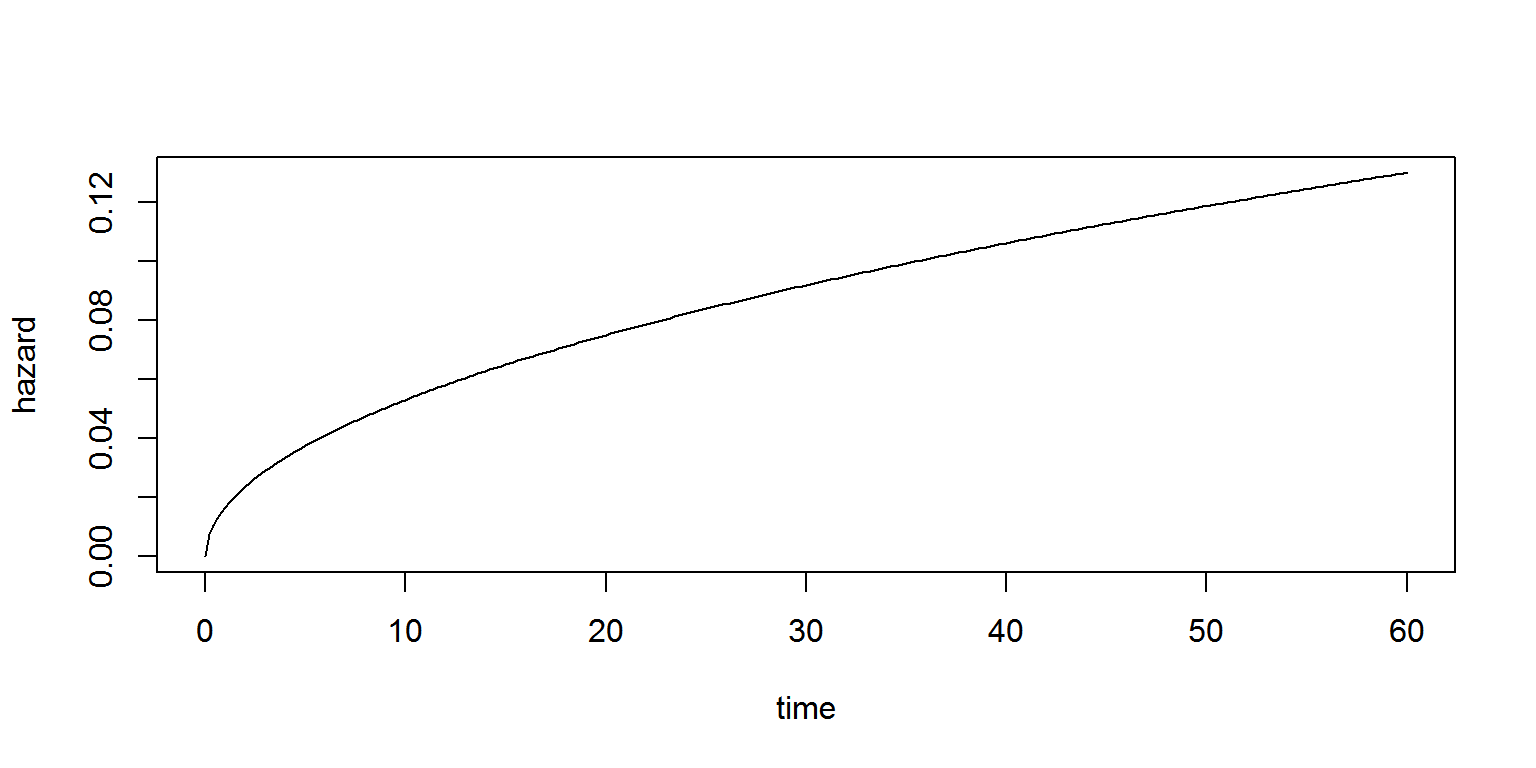We can use the same model for generating 100 single events. An additional argument group is then necessary, with the number of subjects defined in the field size.

N <- 100
res <- simulx(model     = 'model/survival1.txt',
settings  = list(seed=1234),
parameter = p,
output    = list(h, e),
group     = list(size = N))

Some events are exactly observed (e=1) some others are right censored (time=60 and e=0)

print(res$e[1:10,]) ## id time e ## 1 1 0.000000 0 ## 2 1 29.435794 1 ## 3 2 0.000000 0 ## 4 2 10.723412 1 ## 5 3 0.000000 0 ## 6 3 8.983183 1 ## 7 4 0.000000 0 ## 8 4 4.954004 1 ## 9 5 0.000000 0 ## 10 5 4.683973 1 We can now use kmplotmlx for computing and plotting the Kaplan Meier estimate of the survival function with a $$90\%$$ confidence interval: pl1 <- kmplotmlx(res$e, level=0.9)
print(pl1)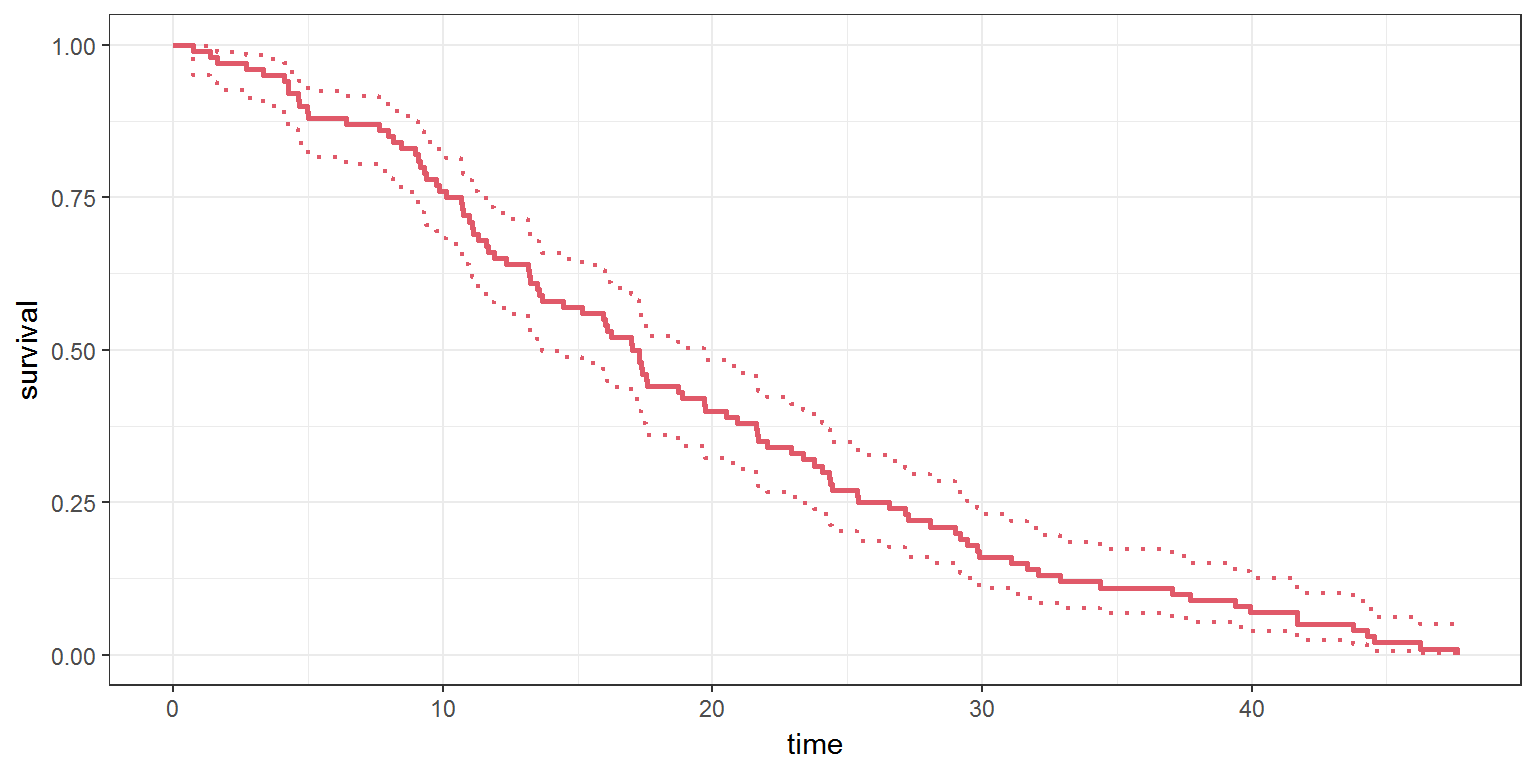# 3 Simulation of repeated events

Assume now that we have repeated events which are interval censored. The length of the intervals is 5.

This new model is implemented in the file survival2.txt:

[LONGITUDINAL]
input = {beta,lambda}

EQUATION:
h=(beta/lambda)*(t/lambda)^(beta-1)

DEFINITION:
e = {type               = event,
eventType          = intervalCensored,
intervalLength     = 5,
rightCensoringTime = 60,
hazard             = h}


We can use simulx with this new model:

res <- simulx(model     = 'model/survival2.txt',
settings  = list(seed=123),
parameter = p,
output    = list(e)) 

res$e contains now the number events in each interval of length 5 between time 0 and time 60. print(res$e)
##    time e
## 1     0 0
## 2     5 0
## 3    10 0
## 4    15 0
## 5    20 0
## 6    25 0
## 7    30 1
## 8    35 0
## 9    40 0
## 10   45 0
## 11   50 0
## 12   55 0
## 13   60 1

We can, as previously, simulate 100 replicates of the same experiment and display the Kaplan Meier plot:

res <- simulx(model     = 'model/survival2.txt',
settings  = list(seed=123),
parameter = p,
output    = list(e),
group     = list(size=N))

pl2  <- kmplotmlx(res$e, level=0.9) print(pl2)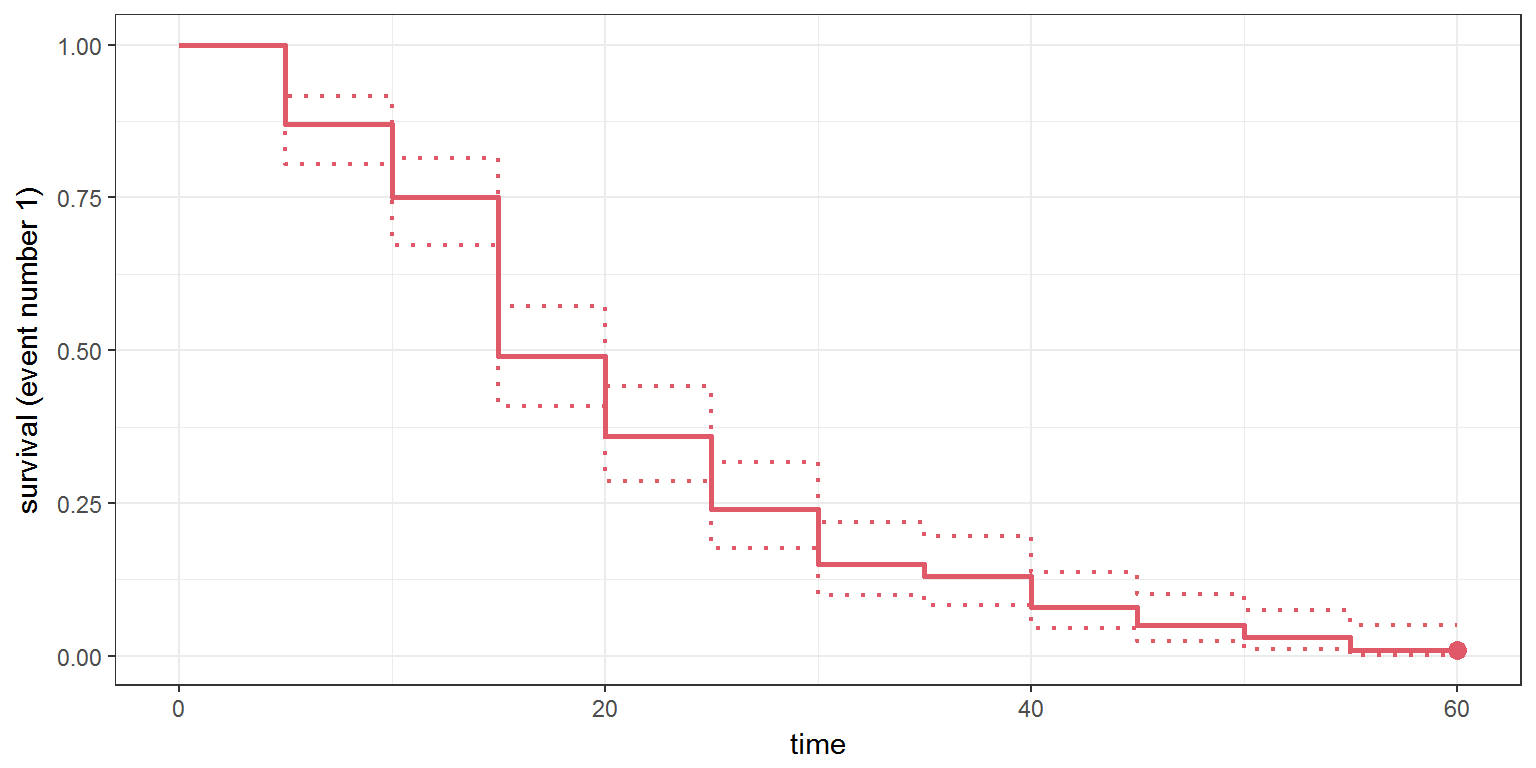# 4 Defining individual designs ## 4.1 Individual right censoring times The right censoring time can be defined per individual tteModel1 <- inlineModel(" [LONGITUDINAL] input = {beta, lambda, rct} EQUATION: h=(beta/lambda)*(t/lambda)^(beta-1) DEFINITION: e = {type=event, maxEventNumber=1, rightCensoringTime=rct, hazard=h} ") N <- 100 p1 <- c(beta=2.5,lambda=50) rct <- data.frame(id=1:N, rct=c(rep(50,N/4),rep(60,N/4),rep(70,N/2))) e <- list(name='e', time=0) res1a <- simulx(model = tteModel1, parameter = list(p1, rct), output = e, settings = list(seed=12345)) print(res1a$e[c(1:4,69:72,173:176),])
##     id      time e
## 1    1  0.000000 0
## 2    1 50.000000 0
## 3    2  0.000000 0
## 4    2  9.873987 1
## 69  35  0.000000 0
## 70  35 36.448000 1
## 71  36  0.000000 0
## 72  36 60.000000 0
## 173 87  0.000000 0
## 174 87 70.000000 0
## 175 88  0.000000 0
## 176 88 39.908235 1
kmplotmlx(res1a$e)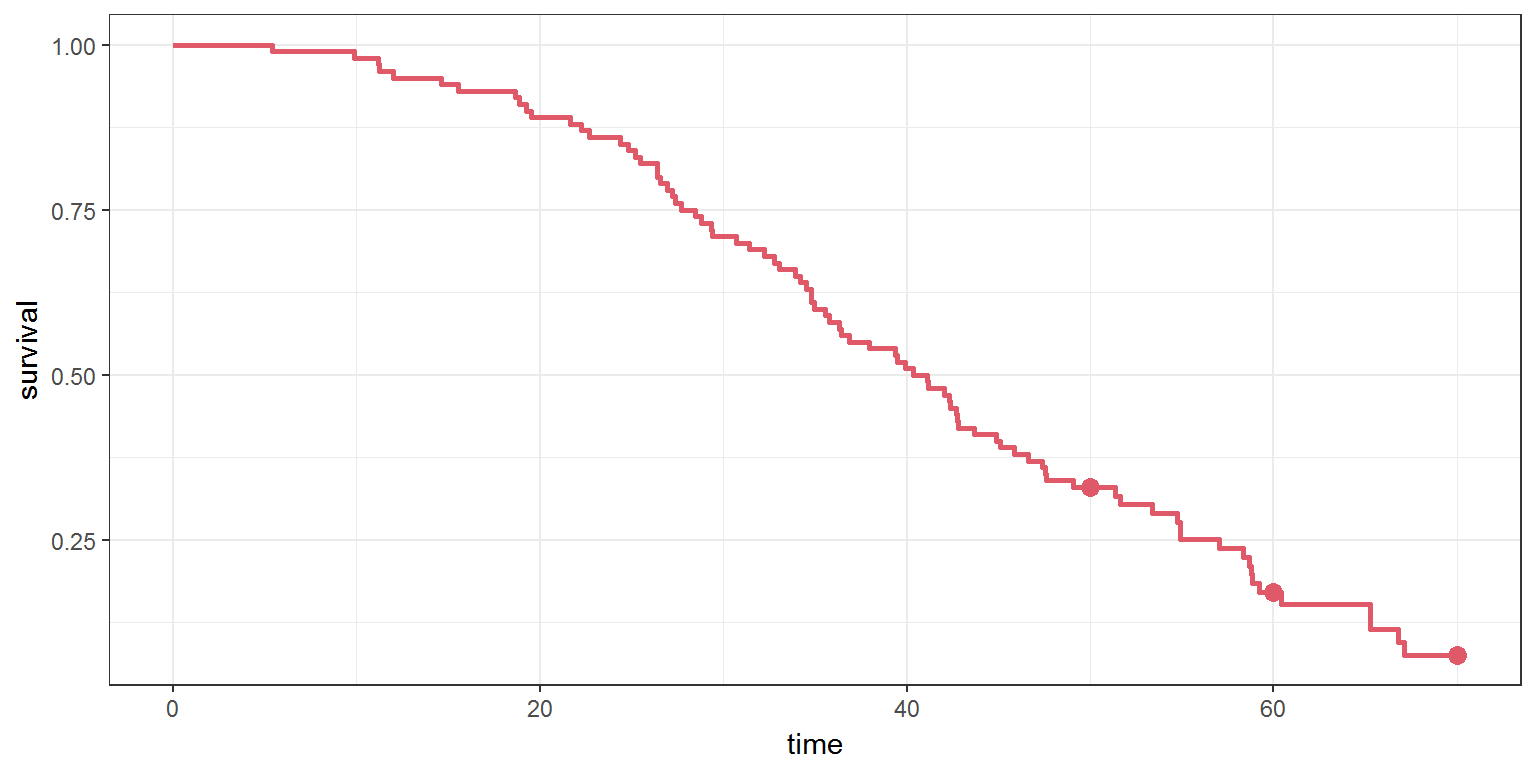or per group g1 <- list(size=50, parameter=c(beta=2.5,lambda=50, rct=60)) g2 <- list(size=100, parameter=c(beta=1,lambda=40, rct=70)) res1b <- simulx(model = tteModel1, output = e, group = list(g1,g2), settings = list(seed=12345)) kmplotmlx(res1b$e)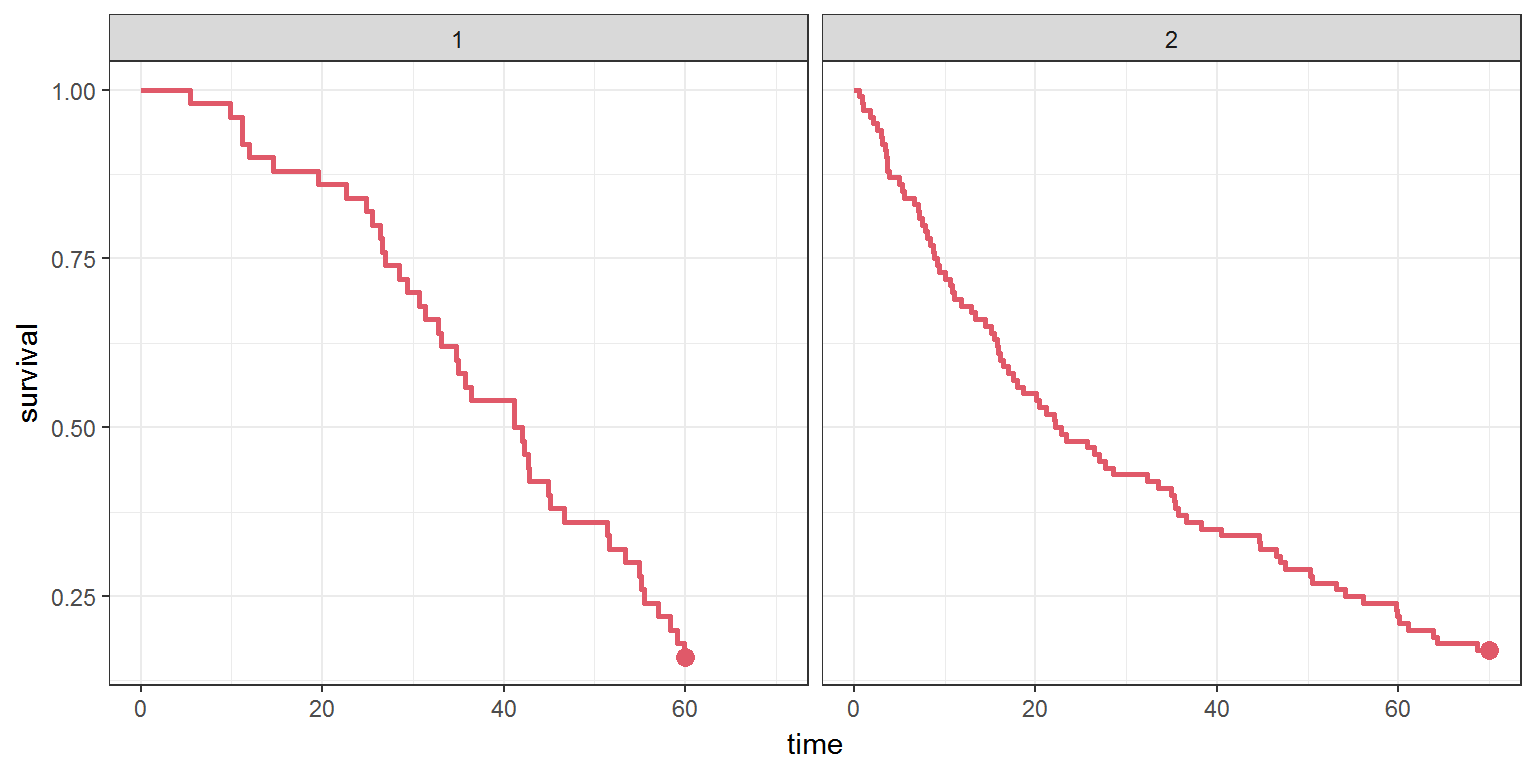## 4.2 Maximum number of events

tteModel1b <- inlineModel("
[LONGITUDINAL]
input = {beta, lambda, men}

EQUATION:
h=(beta/lambda)*(t/lambda)^(beta-1)

DEFINITION:
e = {type=event, maxEventNumber=men, rightCensoringTime=100, hazard=h}
")

N <- 3
p1   <- c(beta=2.5,lambda=50)
men  <- data.frame(id=1:3, men=c(1,2,3)) # individual maximum number of events
e    <- list(name='e', time=0)
res1a <- simulx(model     = tteModel1b,
parameter = list(p1, men),
output    = e,
settings  = list(seed=12345))
print(res1a\$e)
##   id       time e
## 1  1   0.000000 0
## 2  1  59.090342 1
## 3  2   0.000000 0
## 4  2   9.873994 1
## 5  2  53.763458 1
## 6  3   0.000000 0
## 7  3  55.145929 1
## 8  3  99.157858 1
## 9  3 100.000000 0